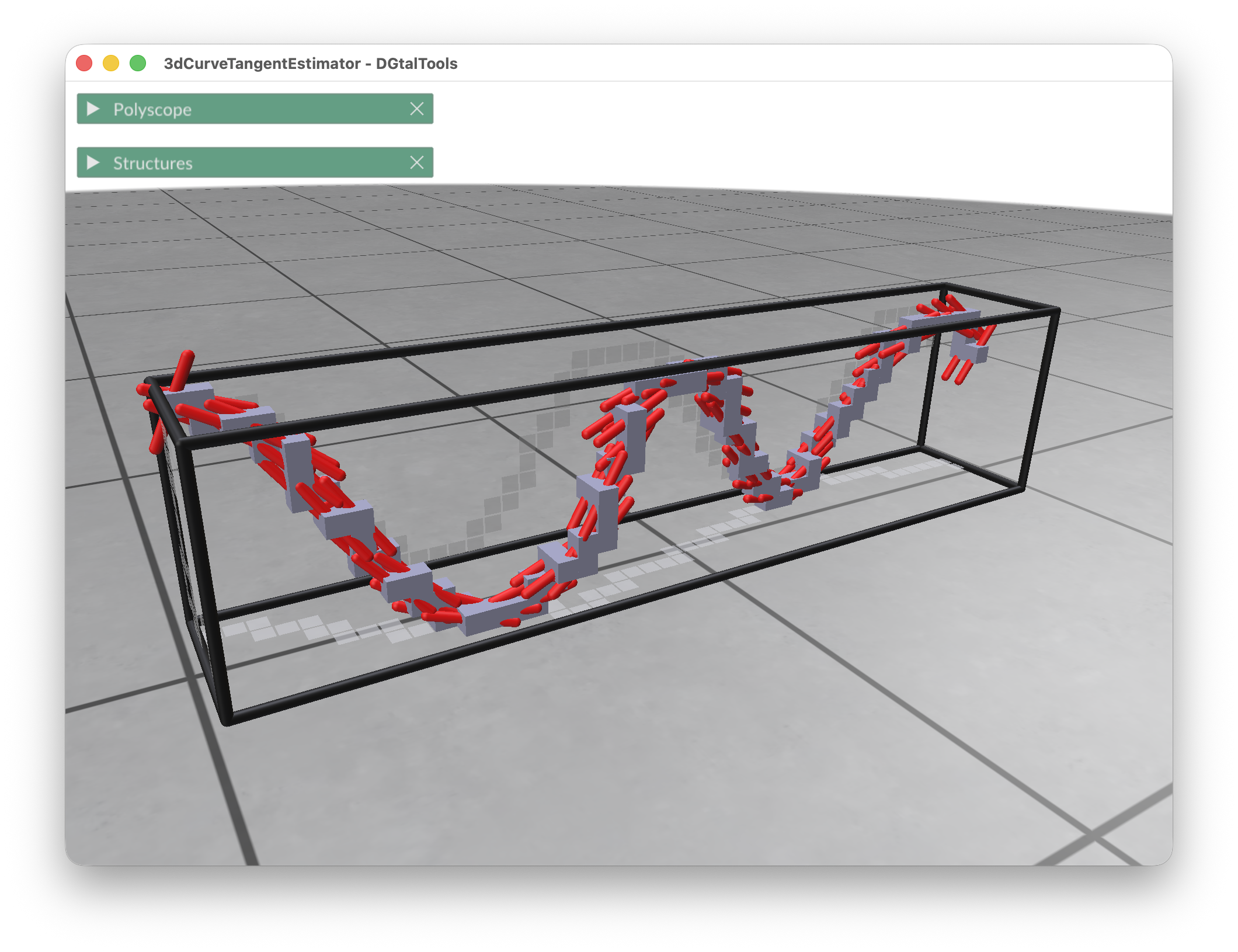DGtalTools  1.3.beta
3dCurveTangentEstimator

This program estimates the tangent vector to a set of 3D integer points, which are supposed to approximate a 3D curve.

Usage: ./estimators/3dCurveTangentEstimator [options] –input <filename>

This program estimates the tangent vector to a set of 3D integer points, which are supposed to approximate a 3D curve. This set of points is given as a list of points in file <input>. The tangent estimator uses either the digital Voronoi Covariance Measure (VCM) or the 3D lambda-Maximal Segment Tangent (L-MST). This program can also displays the curve and tangent estimations, and it can also extract maximal digital straight segments (2D and 3D).

Note
It is not compulsory for the points to be ordered in sequence, except if you wish to compute maximal digital straight segments. In this case, you can select the connectivity of your curve between 6 (standard) or 26 (naive).

Allowed options are :

Positionals:
1 TEXT:FILE REQUIRED the name of the text file containing the list of 3D points: (x y z) per line.
Options:
-h,--help Print this help message and exit
-i,--input TEXT:FILE REQUIRED the name of the text file containing the list of 3D points: (x y z) per line.
-V,--view TEXT=OFF toggles display ON/OFF
-b,--box INT=0 specifies the tightness of the bounding box around the curve with a given integer displacement <arg> to enlarge it (0 is tight)
-v,--viewBox TEXT:{WIRED,COLORED}=WIRED
displays the bounding box, <arg>=WIRED means that only edges are displayed, <arg>=COLORED adds colors for planes (XY is red, XZ green, YZ, blue).
-T,--connectivity TEXT:{6,26}=6 specifies whether it is a 6-connected curve or a 26-connected curve: arg=6 | 26.
-C,--curve3d displays the 3D curve
-c,--curve2d displays the 2D projections of the 3D curve on the bounding box
-3,--cover3d displays the 3D tangential cover of the curve
-2,--cover2d displays the 2D projections of the 3D tangential cover of the curve
-t,--tangent displays the tangents to the curve.
-n,--nbColors UINT=3 sets the number of successive colors used for displaying 2d and 3d maximal segments (default is 3: red, green, blue)
-R,--big-radius FLOAT=10 the radius parameter R in the VCM estimator.
-r,--small-radius FLOAT=3 the radius parameter r in the VCM estimator.
-m,--method TEXT:{VCM,L-MST}=VCM the method of tangent computation: VCM (default), L-MST.
-a,--axes TEXT:{ON,OFF}=OFF show main axes - prints list of axes for each point and color points color = (if X => 255, if Y => 255, if Z => 255)
-o,--output TEXT=3d-curve-tangent-estimations
the basename of the output text file which will contain points and tangent vectors: (x y z tx ty tz) per line

Example: This command line show an example of tangent estimation with the VCM estimator.

3dCurveTangentEstimator \${DGtal}/examples/samples/sinus.dat -V ON -c -R 20 -r 3 -T 6

You should obtain such a result:Resulting tangent vectors (red) obtained with the VCM estimator.